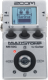Atmosferic Space

Discussion in 'Zoom MS-50G' started by pedromg19, Apr 14, 2019.

1. Atmosferic SpaceDevice: Zoom Ms-50g
Firmware: 3.00

Name on device: CleanSpace
Optimized for: Phones/Speaker

Effects chain:Nice spacious ambient sound. Try messing around with the ice delay interval, my favourites are +-Oct, +-P5 and +-P4.

Effect: "TW ROCK" (Amp simulator), active - "yes"
"Gain" = 0
"Tube" = 0
"Level" = 120
"Trebl" = 60
"Middl" = 60
"Bass" = 20
"Prese" = 90
"CAB" = ​
"OUT" = LINE

Effect: "ICE Delay" (Delay / Reverb), active - "yes"
"TIME" = 787
"F.B" = 100
"MIX" = 48
"INTVL" = +Oct
"SLICE" = SHORT
"BLEND" = 8
"SMEAR" = 11
"DAMP" = 2
"HPF" = 230

Effect: "HD Reverb" (Delay / Reverb), active - "yes"
"Decay" = 90
"Tone" = 5
"Mix" = 89
"PreD" = 71
"HPF" = 7
"Level" = 80
"Tail" = Off

Effect: "ParaEQ" (Dynamics / Filter), active - "yes"
"Freq1" = 320Hz
"Q1" = 8
"Gain1" = -6
"Freq2" = 1.2kHz
"Q2" = 0.5
"Gain2" = 6
"Level" = 60

Note: This is a patch file, you will need to download and install the ToneLib-Zoom software to use the patch.

File size:
408 bytes
Views:
68